# Crystal Ball[Page 634 ( continued )]

So far in this chapter we have used simulation examples that included mostly discrete probability distributions that we set up on an Excel spreadsheet. These are the easiest types of probability distributions to work with in spreadsheets. However, many realistic problems contain more complex probability distributions, like the normal distribution, which are not discrete but are continuous, or they include discrete probability distributions that are more difficult to work with than the simple ones we have used. However, there are several simulation add-ins for Excel that provide the user with the capability to perform simulation analysis, using a variety of different probability distributions in a spreadsheet environment. One of these add-ins is Crystal Ball, published by Decisioneering; it is available for download from the CD that accompanies this text. Crystal Ball is a risk analysis and forecasting program that uses Monte Carlo simulation to forecast a statistical range of results possible for a given situation. In this section we will provide an overview of how to apply Crystal Ball to a simple example for profit analysis that we first introduced in Chapter 1.

#### Simulation of a Profit Analysis Model

In Chapter 1 we used a simple example for the Western Clothing Company to demonstrate break-even and profit analysis. In that example, Western Clothing Company produced denim jeans . The price ( p ) for jeans was \$23, the variable cost ( c v ) was \$8 per pair of jeans, and the fixed cost ( c f ) was \$10,000. Given these parameters, we formulated a profit ( Z ) function as follows :

Z = vp c f vc v

Our objective in that analysis was to determine the break-even volume, v , that would result in no profit or loss. This was accomplished by setting Z = 0 and solving the profit function for v , as follows: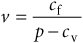[Page 635]

Substituting the values for p , c f , and c v into this formula resulted in the break-even volume: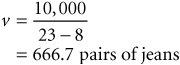To demonstrate the use of Crystal Ball, we will modify that example. First, we will assume that volume is actually volume demanded and that it is a random variable defined by a normal probability distribution, with a mean value of 1,050 pairs of jeans and a standard deviation of 410.

Furthermore, we will assume that the price is not fixed but is also uncertain and defined by a uniform probability distribution (from \$20 to \$26) and that variable cost is not a constant value but defined by a triangular probability distribution. Instead of seeking to determine the break-even volume, we will simulate the profit model, given probabilistic demand, price, and variable costs to determine average profit and the probability that Western Clothing will break even.

The first thing we need to do is access Crystal Ball, which you can download from the CD that comes with this text.

Exhibit 14.12 shows the Excel spreadsheet for our example.  We have described the parameters of each probability distribution in our profit model next to its corresponding cell. For example, cell C4 contains the probability distribution for demand. What we want to do is generate demand values in this cell according to the probability distribution for demand (i.e., Monte Carlo simulation). We also want to do this in cell C5 for price and in cell C7 for variable cost. This is the same process we used in our earlier ComputerWorld example to generate demand values from a discrete probability distribution, using random numbers . Notice that cell C9 contains our formula for profit, = C4*C5-C6-(C4*C7) . This is the only cell formula in our spreadsheet.

 This simulation example is located on the CD accompanying this text. It is in a file titled "Exhibit 14.12," located in the Crystal Ball folder on the accompanying text CD and created when Crystal Ball is loaded from the CD.

##### Exhibit 14.12.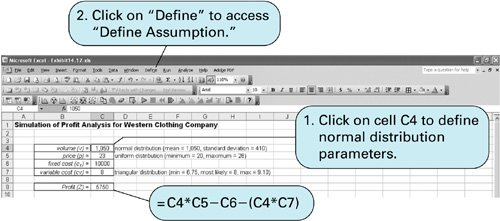To set up the normal probability distribution for demand, we first enter the mean value 1,050 in cell C4. Cells require some initial value to start with. Next we click on "Define" from the top of the spreadsheet, as shown in Exhibit 14.12, which will result in the menu shown in Exhibit 14.13. We select "Define Assumption" from this menu, which will result in the Distribution Gallery window shown in Exhibit 14.14.

[Page 636]
##### Exhibit 14.13.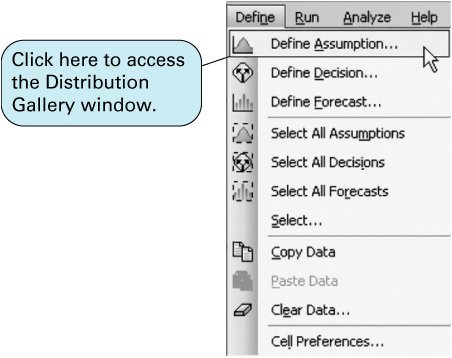##### Exhibit 14.14.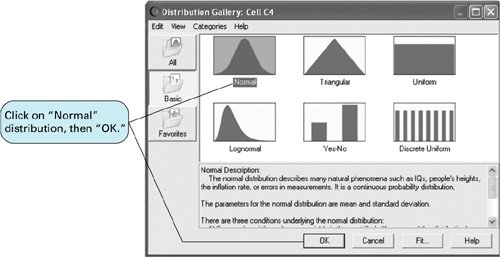The Distribution Gallery window includes several different probability distributions we can use. Because we have indicated that demand is defined by a normal distribution, we click on this box and then on "OK." This will result in the window for the normal distribution shown in Exhibit 14.15.

##### (This item is displayed on page 637 in the print version)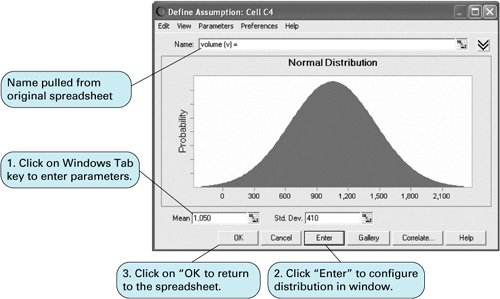The "Name" value in the box at the top of the window in Exhibit 14.15 was automatically pulled from the spreadsheet, where it is the heading "volume (v) ="; however, a new or different name could be typed in. Next, we click on "Mean" or use the Tab key to toggle down to the "Mean" display in the lower-left-hand corner of this window. Because we entered the mean value of 1,050 in cell C4 on our spreadsheet, this value will already be shown in this window. Next, we click on "Std Dev" or use the Tab key to move to the "Std Dev" window and enter the standard deviation of 410. Then we click on the Enter button, which will configure the normal distribution figure in the window, and then we click on "OK."

[Page 637]

We will repeat this same process to enter the parameters for the uniform distribution for price in cell C5. First, we enter the value for price, 23, in cell C5. Next (with cell C5 activated), we click on "Define" at the top of the spreadsheet and then select "Define Assumption" from the menu, as shown earlier in Exhibit 14.13. The Distribution Gallery window will again appear, and this time you should click on "Uniform Distribution" and then "OK." This will result in the "Uniform Distribution" window shown in Exhibit 14.16.

##### Exhibit 14.16.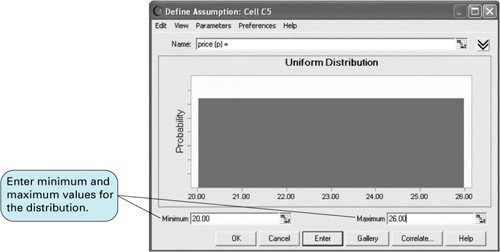[Page 638]

As before, the "Name" value, "price (p)," was pulled from the original spreadsheet in Exhibit 14.12. Next, we click on "Minimum" or use the Tab key to move to the "Minimum" display at the bottom of the window and enter 20, the lower limit of the uniform distribution specified in the problem statement. Next, we activate the "Maximum" display window and enter 26. Then we click on the "Enter" button to configure the distribution graph in the window. Finally, we click on "OK" to exit this window.

We repeat the same process to enter the triangular distribution parameters in cell C7. A triangular probability distribution is defined by three estimated valuesa minimum, a most likely, and a maximum. It is a very useful approximation when enough data points do not exist to allow for the construction of a distribution, but the user can estimate what the endpoints and the midpoint of the distribution might be. Clicking on "Define Assumption" from the cell menu and then selecting the triangular distribution from the distribution gallery results in the window shown in Exhibit 14.17.

##### Exhibit 14.17.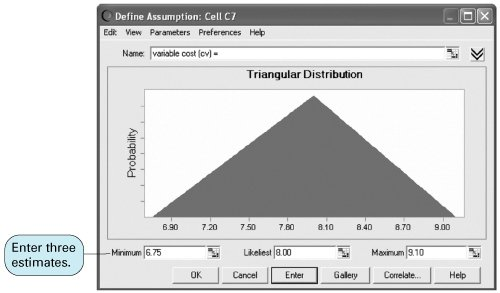We enter the "Minimum" value of 6.75, the "Likeliest" value of 8.00, and the "Maximum" value of 9.10. Clicking on "Enter" will configure the graph of the triangular distribution shown in the window. We click on "OK" to exit this window and return to the spreadsheet.

Next, we click on cell C9 on our original spreadsheet. Recall that this is the cell in which we entered our profit formula in Exhibit 14.12. The profit value of 5,750, computed from the other cell values entered on the original spreadsheet, will be shown in cell C9. We click on the "Define" menu at the top of the spreadsheet and select "Define Forecast," as shown in Exhibit 14.18. This will result in the window shown in Exhibit 14.19. The heading "Profit(Z) =" will already be entered from the spreadsheet. We click on the "Units" display and enter "dollars." We then click on "OK" to exit this window. This completes the process of entering our simulation parameters and data. Exhibit 14.20 shows the spreadsheet with changes resulting from the parameter inputs. The next step is to run the simulation.

##### (This item is displayed on page 639 in the print version)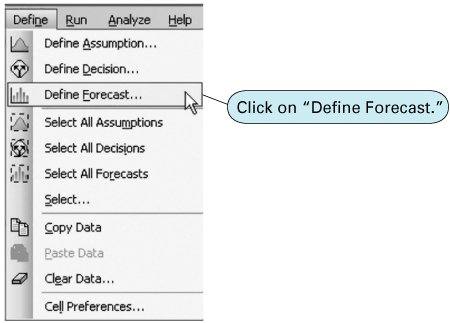##### (This item is displayed on page 639 in the print version)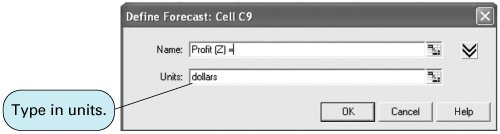##### (This item is displayed on page 639 in the print version)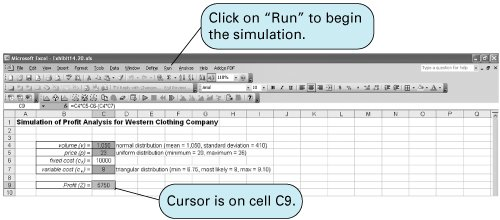The mechanics of the simulation are similar to those of our previous Excel spreadsheet models. Using random numbers, we want to generate a value for demand in cell C4, then a value for price in C5, and then a value for variable cost in C7. These three values are then substituted into the profit formula in cell C9 to compute a profit value. This represents one repetition, or trial , of the simulation. The simulation is run for many trials in order to develop a distribution for profit.

[Page 639]

To run the simulation, we access the "Run" menu at the top of the spreadsheet. This will result in the menu shown in Exhibit 14.21. We click on "Run Preferences," which will activate the window shown in Exhibit 14.22. We then enter the number of simulations for the simulation run. For this example we will run the simulation for 5,000 trials. Next, we click on "Sampling" at the top of this window to activate the window shown in Exhibit 14.23. In this window we must enter the seed value for a sequence of random numbers for the simulation, which is always 999. We click on "OK" and then go back to the "Run" menu. From the "Run" menu (Exhibit 14.21), we click on "Start Simulation," which will run the simulation. Exhibit 14.24 shows the simulation window with the simulation completed for 5,000 trials and the frequency distribution for this simulation.

[Page 641]
##### (This item is displayed on page 640 in the print version)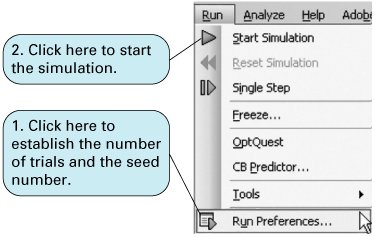##### (This item is displayed on page 640 in the print version)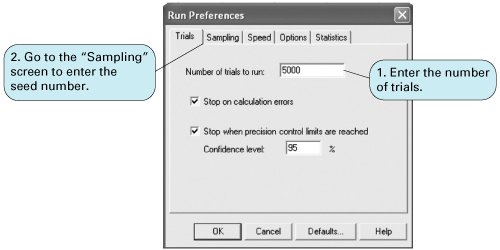##### (This item is displayed on page 640 in the print version)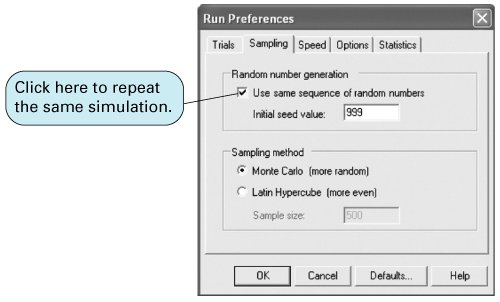##### Exhibit 14.24.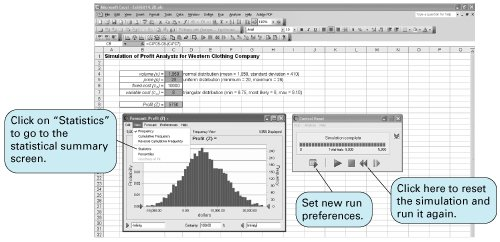A statistical summary report for this simulation can be obtained by clicking on "View" at the top of the forecast window and then selecting "Statistics" from the drop-down menu. This results in the window shown in Exhibit 14.25. You can return to the forecast window by selecting "Frequency" from the "View" menu at the top of the statistics window.

In our original example formulated in Chapter 1, we wanted to determine the break-even volume. In this revised example, Western Clothing Company wants to know the average profit and the probability that it will break even from this simulation analysis. The mean profit (from the Statistics window in Exhibit 14.25) is \$5,833.78. The probability of breaking even is determined by clicking on the arrow on the left side of the horizontal axis of the window shown in Exhibit 14.26 and " grabbing " it and moving it to "0.00," or by clicking on the lower limit, currently set at "Infinity"; we change this to 0 and press the Enter key. This will shift the lower limit to zero, the break-even point. The frequency chart that shows the location of the new lower limit and the "Certainty" of zero profit is shown as 81.73% at the bottom of the window as shown in Exhibit 14.26. Thus, there is a .8173 probability that the company will break even.

##### (This item is displayed on page 642 in the print version)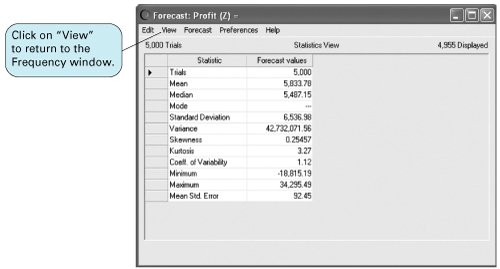##### (This item is displayed on page 642 in the print version)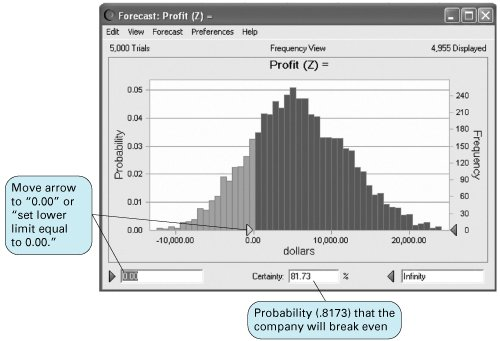We have demonstrated using Crystal Ball with a straightforward example that was not very complex or detailed. Crystal Ball has the capability to perform much more sophisticated simulation analyses than what we have shown in this section. However, the demonstration of these capabilities and other features of Crystal Ball would require more space and in-depth coverage than is possible here. However, although using Crystal Ball to simulate more complex situations requires a greater degree of knowledge than we have provided, this basic introduction to and demonstration of Crystal Ball provide a good starting point to understanding the basic features of Crystal Ball and its use for simulation analysis.Introduction to Management Science (10th Edition)
ISBN: 0136064361
EAN: 2147483647
Year: 2006
Pages: 358

Similar book on Amazon

flylib.com © 2008-2017.
If you may any questions please contact us: flylib@qtcs.net